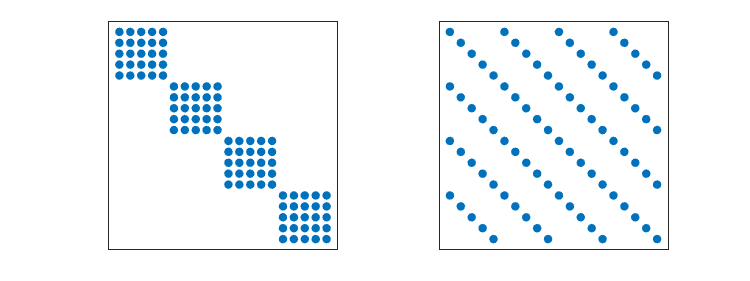# Two Dubious Ways to Solve A*X = X*B, part 1

Recently, I had email from a student in Italy.

I am doing a project on Hand-Eye calibration. I have found A, the transformation from camera to world, and B, the transformation from gripper to base. I want to find the transformation from gripper to camera. This is the solution of the equation AX = XB. Can you me any advice for solving this equation?

Later, my correspondent found the solution himself; his matrices were only 4-by-4 and there is an analytic solution using quaternions.

But the general question stuck with me. How do you solve A*X = X*B? The equation is ill-posed. In part 2, I will describe two methods for solving it. Both methods have serious drawbacks. First, some background.

### Contents

#### Inhomogeneous Sylvester Equation

The standard, inhomogeneous, Sylvester equation involves three nonzero matrices, A, B, and C.

A*X + X*B = C

This equation is fundamental in control theory and the function sylvester(A,B,C) has been part of MATLAB for many years. The help entry for sylvester does not mention any conditions, other than compatible dimensions, on A, B, and C. Near the end of the documentation for sylvester is this single sentence:

The equation has a unique solution when the eigenvalues of A and -B are distinct.

If we deliberately violate this condition by taking B to be -A', we find that the computed solution blows up. Let's see this with the matrix

A = gallery(3)

A =

   -149   -50  -154
537   180   546
-27    -9   -25

It isn't obvious, but this matrix was constructed so that its eigenvalues are 1, 2, and 3.

e = eig(A)

e =

   1.0000
2.0000
3.0000

Now solve the Sylvester equation, deliberately violating the condition that A and -B not have any eigenvalues in common.

X = sylvester(A,-A',eye(3))


The solution wants to be infinite, but roundoff gives us 1/eps.

X =

   1.0e+15 *
   -0.8519    1.1016    0.4883
1.1016   -1.4822   -0.6330
0.4883   -0.6330   -0.2726

I will take advantage of this behavior one of the methods.

#### Homogeneous Sylvester Equation

If I take C to be zero, and flip the sign of B, the Sylvester equation becomes

(1) A*X = X*B

This is our homogeneous Sylvester equation and I've labeled it (1). It turns out that (1) has a nonzero solution if and only if A and B have at least one eigenvalue in common. Nothing is required of the rank of the solution X, but it is clear that this rank cannot exceed the number of eigenvalues that A and B have in common.

Equation (1) is a linear equation. If X and Y are solutions, so is alpha*X + beta*Y for any scalars alpha and beta.

In order to have compatible dimensions, A and B have to be square, but they do not have to be the same size. If A is m-by-m and B is n-by-n, then X is m-by-n .

#### Moler's Laws

My fundamental Laws of Scientific Computing are:

• The hardest things in the world to compute are things that do not exist.
• The next hardest are things that are not unique.

Problems which fail to have unique solutions are ill-posed. Even when solutions exist, they are wildly sensitive to perturbations.

Equation (1) is a poster child for ill-posed. Without restrictions on A and B, the only solution is zero. On the other hand, if A and B share at least one eigenvalue, there is at least one solution, but it is not unique because it can be renormalized. And the number of linearly independent solutions depends upon elusive algebraic and geometric multiplicities.

#### B = 0

Let's look at some special versions of (1). If we were to write some general purpose software, it will have to deal with all these cases.

If B is zero, (1) becomes A*X = 0 and any vector in the null space of A is a solution. The best way to find the null space of a matrix uses its SVD.

#### B is diagonal

If B is diagonal with scalar elements d, then (1) becomes A*x = d*x. There are solutions if d is an eigenvalue, but they aren't unique.

#### B = A'

Any matrix and its transpose have the same eigenvalues, so the equation becomes

(2) A*X = X*A'

This is the most common form of the homogeneous Sylvester equation. If the solution has full rank, then X\A*X = A' and we are asking when is a matrix similar to its transpose.

If A has a full set of linearly independent eigenvectors, then so does A'. If U\A*U = D = V\A'*V where D is diagonal, then X = V/U is a solution to (2) .

If A = J is a Jordan block for any eigenvalue, let X = fliplr(eye(n)) . Then X is a solution to (2) .

#### A is symmetric

If A = A', (2) has many solutions, including the identity matrix, any other polynomial in A, and even functions like exp(t*A).

#### Preview of part 2

That's enough for now. In part two, I will investigate two methods, one using Kronecker products and another using inverse iteration. Here's a preview of the Kronecker products.#### Further reading

An article by Nick Higham about the sensitivity of the Sylvester equation. link.

A paper by Olga Taussky and Hans Zassenhuas about when the solution of X\A*X = A' is symmetric. link.

A paper and Fortran software by Alan Laub, me, and three of our students for A*X*B' + C*X*D' = E. link, link.

Published with MATLAB® R2020b

|

### Comments

To leave a comment, please click here to sign in to your MathWorks Account or create a new one.